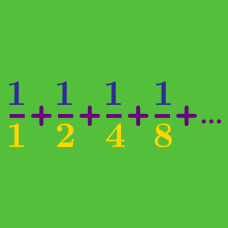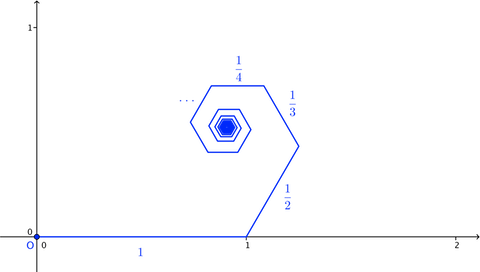Calculus

# Sequences and Series: Level 4 Challenges

$\large \frac ab \ , \ ab \ , \ a -b \ , \ a+b$

Above shows real numbers that belong to an arithmetic progression in order. Find the next term of this sequence.

Find the number of $6$-term strictly increasing geometric progressions, such that all terms are positive integers less than $1000.$

${2^x + 2^{x+1} + 2^{x+2} + \ldots + 2^{x+2015} = 4^x + 4^{x+1} + 4^{x+2} + \ldots + 4^{x+2015}}$

If $x$ satisfies the equation above and it can be represented as $\log_D \left(\dfrac{A}{1+B^C} \right)$ for positive integers $A$, $B$, $C$, and $D$, where $B$ is prime, determine the smallest value of $A + B + C +D$.The image above shows a broken line (a series of connected line segments) starting at the origin, O. The nth segment in the broken line has length $\frac{1}{n}$, and at the end of each segment, the broken line turns $60^{\circ}$ counter-clockwise.

As the number of segments in the broken line approaches infinity, the final endpoint of the broken line approaches a point P. The distance OP can be written as $\frac{a}{b}\pi$, where a and b are positive coprime integers. Find $a+b$.

$\cot^{-1} 3 + \cot^{-1} 7 + \cot^{-1} 13 + \cot^{-1} 21 + \cot ^ {-1} 31 + \ldots$

Evaluate the sum above in degrees.

×